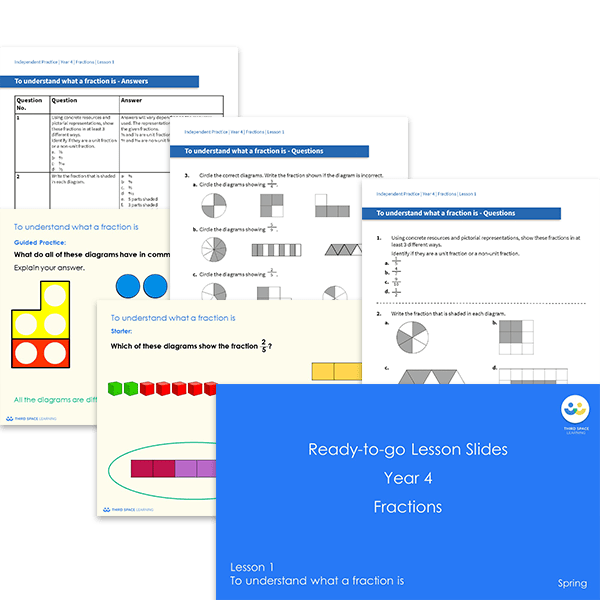# Ready-to-go lessons Year 4 Fractions (Spring Block 3) Slides and Worksheets

Fractions ready-to-go lessons for Year 4 includes 10 PowerPoints with matching worksheets ready for you to use with a class. These resources follow a mastery approach to mathematics teaching, similar to schemes such as White Rose Maths and Mathematics Mastery. The PowerPoints are fully editable with detailed notes for every teaching slide. Each associated worksheet is divided into three parts with a range of activities to complete across the block.

This Year 4 Fractions pack contains 10 fully editable PowerPoints and 10 worksheets.

The slides and worksheets cover:

• Understanding fractions
• Finding and showing equivalent fractions
• Understanding and showing fractions greater than one
• Counting in fractions greater than one
• Adding two or more fractions
• Subtracting fractions (including from a whole number)
• Finding a fraction of a quantity
• Using fractions to calculate quantities

Year 4

Fractions